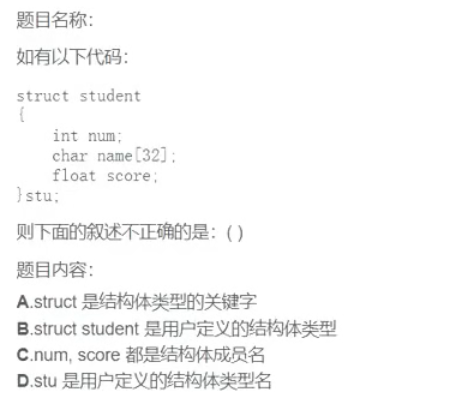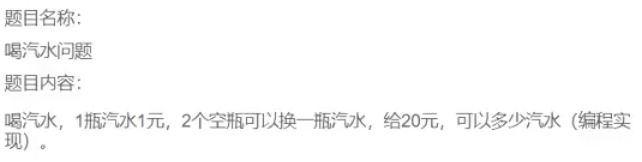结构体定义形式：

``````struct 结构体名
{
成员列表（可以是基本数据类型、指针、数组或其他结构类型）;
}``````

struct是结构体关键字，struct+结构体名 是结构体类型，stu是通过上面的结构体类型定义的结构体变量。``````int main()
{
int empty = 0;  //空瓶
int money = 20;  //钱数
int total = 0;  //总数
empty = money / 1;
total = empty;
while (empty >= 2)
{
total +=empty / 2;
empty = empty % 2 + empty / 2;
}
printf("%d", total);  //39
return 0;
}``````Suzhou
Lv1

17

11

0